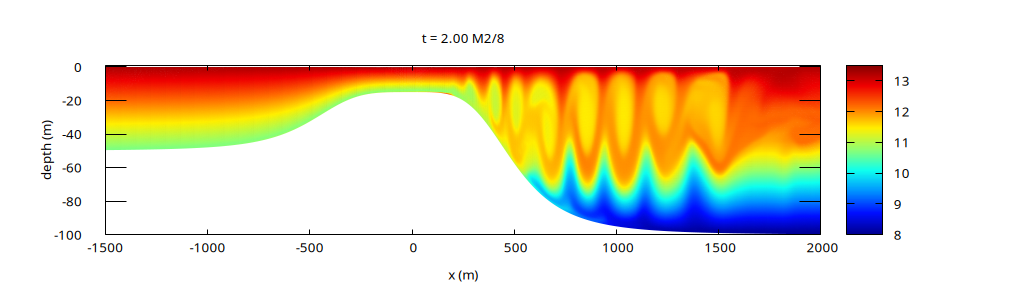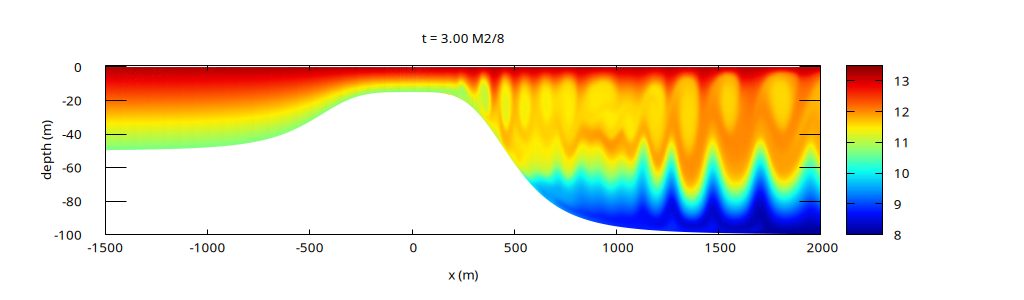# Tidally-induced internal lee waves

This example illustrates the importance of non-hydrostatic effects for the generation of internal waves by barotropic tides flowing over a sill, as first studied by Xing & Davies, 2007.

A close up of the full domain close to the sill is illustrated in the movie below. The sill geometry (hidden in Berntsen et al, 2009) is given by \displaystyle \begin{aligned} H(x) & = -50 + \frac{35}{1+(x/500)^4} & \text{ if }x < 0 \\ H(x) & = -100 + \frac{85}{1+(x/500)^4} & \text{ if }x > 0 \\ \end{aligned} The barotropic tide is imposed as inflow on the left boundary. Breaking, non-hydrostatic internal waves are generated on the lee side.

Evolution of the temperature field

The results at t=2/8 T and t = 3/8 T below, with T the M2 tidal period, can be compared, for example, with the corresponding results of Klingbeil & Burchard, 2013, Figure 9, a1 and b1.Temperature field at t=2/8 TTemperature field at t=3/8 T

 [klingbeil2013] Knut Klingbeil and Hans Burchard. Implementation of a direct nonhydrostatic pressure gradient discretisation into a layered ocean model. Ocean Modelling, 65:64 – 77, 2013. [ DOI | http ] [berntsen2009] Jarle Berntsen, Jiuxing Xing, and Alan M Davies. Numerical studies of flow over a sill: sensitivity of the non-hydrostatic effects to the grid size. Ocean Dynamics, 59(6):1043, 2009. [ DOI ] [davies2007] Alan M Davies and Jiuxing Xing. On the influence of stratification and tidal forcing upon mixing in sill regions. Ocean Dynamics, 57(4-5):431–451, 2007. [ DOI ] [xing2007] Jiuxing Xing and Alan M. Davies. On the importance of non-hydrostatic processes in determining tidally induced mixing in sill regions. Continental Shelf Research, 27(16):2162 – 2185, 2007. [ DOI | http ]

## Code

We use the 1D (horizontal) non-hydrostatic multilayer solver.

#include "grid/multigrid1D.h"
#include "layered/hydro.h"
#include "layered/nh.h"

The Boussinesq density perturbation is given as a function of the “temperature” field T.

#define drho(T) (1e-3*(T - 13.25)/(8. - 13.25))
#define T0(z) (8. + (13.25 - 8.)*(z + 100.)/100.)
#include "layered/dr.h"

The layer positions are remapped to \sigma levels and performance statistics are displayed.

#include "layered/remap.h"
#include "layered/perfs.h"
// #include "profiling.h"

The horizontal viscosity is set to 0.1 m/s2.

double nu_H = 0.1;

int main()
{
L0 = 21500;
X0 = - 6500;
G = 9.81;

100 layers are used and the horizontal resolution of ~10 metres matches that of Klingbeil & Burchard, 2013. Monotonic limiting is used for vertical remapping.

  nl = 100;
N = 2048;
cell_lim = mono_limit;

The maximum timestep is set to 100 seconds. The actual timestep is limited to about 5 seconds due to the CFL condition based on the maximum horizontal velocity and spatial resolution. Note that this is still much larger than the timestep used by Klingbeil & Burchard (0.56 seconds) and Bernsten et al., 2009 (0.3 seconds). An explanation for such a small timestep could be that the CFL restriction due to vertical motions can be quite restrictive for a vertically-Eulerian discretisation. For this (non-hydrostatic) example, the vertical velocities are of the same order as the horizontal velocities and since the vertical resolution is approx. 10 times larger than the horizontal resolution, the vertical CFL criterion is correspondingly smaller. This restriction is avoided with the vertically-Lagrangian solver.

  DT = 100.;

nu = 1e-3;

system ("rm -f plot-*.png"); // fixme: should not be necessary
run();
}

The M2 tidal period (in seconds).

#define M2 (12.*3600. + 25.2*60.)

The temperature profile T0(z) is imposed at inflow. The slightly complicated function below computes the vertical coordinate of a layer and returns the corresponding temperature.

double Tleft (Point point)
{
double zc = zb[];
for (int l = - point.l; l < nl - point.l; l++)
if (l < 0)
zc += h[0,0,l];
zc += h[]/2.;
return T0(zc);
}

The M2 tide with an amplitude of 0.3 m/s is imposed at inflow (left boundary) as well as the temperature profile. The outflow (right boundary) is free.

event init (i = 0)
{
u.n[left]  = dirichlet (0.3*sin(2.*pi*(t/M2)));
u.n[right] = neumann(0.);
h[right] = dirichlet(100./nl);
T[left] = Tleft(point);
T[right] = Tleft(point);

The sill geometry, initial layer depths and initial temperature profile.

  foreach() {
zb[] = x < 0. ?
-50. + 35./(1. + pow(x/500.,4)) :
-100. + 85./(1. + pow(x/500.,4));
double z = zb[];
foreach_layer() {
h[] = - zb[]/nl;
z += h[]/2.;
T[] = T0(z);
z += h[]/2.;
}
}
}

A naive discretisation of the horizontal viscosity.

event viscous_term (i++)
{
if (nu_H > 0.) {
scalar d2u[];
foreach_layer() {
foreach()
d2u[] = (u.x + u.x[-1] - 2.*u.x[])/sq(Delta);
foreach()
u.x[] += dt*nu_H*d2u[];
#if NH
foreach()
d2u[] = (w + w[-1] - 2.*w[])/sq(Delta);
foreach()
w[] += dt*nu_H*d2u[];
#endif // NH
}
}
}

## Outputs

// fixme: plotting is (almost) the same as overflow.c

void setup (FILE * fp)
{
fprintf (fp,
#if ISOPYCNAL
"set pm3d map corners2color c2\n"
#else
"set pm3d map interpolate 2,2\n"
#endif
"# jet colormap\n"
"set palette defined ( 0 0 0 0.5647, 0.125 0 0.05882 1, 0.25"
" 0 0.5647 1, 0.375 0.05882 1 0.9333, 0.5 0.5647 1 0.4392, 0.625"
" 1 0.9333 0, 0.75 1 0.4392 0, 0.875 0.9333 0 0, 1 0.498 0 0 )\n"
"unset key\n"
"set cbrange [8:13.5]\n"
"set xlabel 'x (m)'\n"
"set ylabel 'depth (m)'\n"
"set xrange [-1500:2000]\n"
"set yrange [-100:1]\n"
);
}

void plot (FILE * fp)
{
fprintf (fp,
"set title 't = %.2f M2/8'\n"
"sp '-' u 1:2:4\n",
t/(M2/8.));
foreach (serial) {
double z = zb[];
fprintf (fp, "%g %g %g %g\n", x, z, u.x[], T[]);
foreach_layer() {
z += h[];
fprintf (fp, "%g %g %g %g\n", x, z, u.x[], T[]);
}
fprintf (fp, "\n");
}
fprintf (fp, "e\n\n");
//  fprintf (fp, "pause 1\n");
fflush (fp);
}

event gnuplot (t += M2/1024.)
{
static FILE * fp = popen ("gnuplot 2> /dev/null", "w");
fprintf (fp, "set term x11 size 1024,300\n");
if (i == 0)
setup (fp);
plot (fp);
fprintf (fp,
"set term pngcairo font \",10\" size 1024,300\n"
"set output 'plot-%04d.png'\n"
"replot\n", i);
}

event figures (t <= M2/2.; t += M2/8.)
{
FILE * fp = popen ("gnuplot 2> /dev/null", "w");
fprintf (fp,
"set term pngcairo font \",10\" size 1024,300\n"
"set output 'T-%g.png'\n", t/(M2/8.));
setup (fp);
plot (fp);
}

event moviemaker (t = end)
{
system ("for f in plot-*.png; do convert $f ppm:- && rm -f$f; done | "
"ppm2mp4 movie.mp4");
}